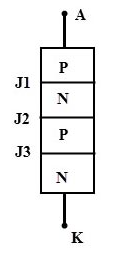Engineering Jobs   »   Electrical Engineering quizs   »   UPRVUNL ELECTRICAL 2021, uprvunl je electrical...

# UPRVUNL’21 EE: Daily Practices Quiz 08-Sep-2021

UPRVUNL JE Recruitment 2021:

A total of 196 vacancies were released by the UPRVUNL Recruitment 2021. The selection process will be based on the written examination which will be divided into two parts – Part I and Part II. Part I will have 150 objective-type questions from the syllabus for the Diploma Engineering in the relevant branch. While Part II will have 50 objective-type questions comprising General Knowledge, General Hindi, and Reasoning.

UPRVUNL’21 EE:  Daily Practices Quiz 08-Sep-2021

Each question carries 1 mark.

Negative marking: 1/4 mark

Total Questions: 06

Time: 08 min

Q1. In a split-phase motor, the running winding should have
(a) High resistance and low inductance
(b) High resistance and High inductance
(c) Low resistance and high inductance
(d) Low resistance and low inductance

Q2. If the area, current, and the number of turns of an AC circuit is doubled, then the new inductance will be: –
(a) Twice the former
(b) Four Times the former
(c) Small
(d) Half the former

Q3. Choose the characteristics which are not related to an ideal OP-AMP.
(a) Very high gain
(b) Very high input impedance
(c) Very low CMRR
(d) Very low output

Q4. Power transformers are designed to have maximum efficiency at

Q5. A silicon-controlled rectifier (SCR) is a
(a) Unijunction device
(b) Device with three junctions
(c) Device with four junctions
(d) None of the above

Q6. A dc series motor drawing an armature current of I_a is operating under saturated magnetic conditions, torque developed in the motor is proportional to
(a) 1/Ia
(b) 1/Ia^2
(c) Ia^2
(d) Ia

SOLUTIONS
S1. Ans.(c)
Sol. split phase motor has two winding one is starting winding and the other is main winding or running winding.
The starting winding has fewer turns and a small diameter; therefore, it has high resistance and low inductance.
The running winding has a heavier wire of more turns; therefore, it has low resistance and high inductance. i.e., running winding is inductive in nature.

S2. Ans.(a)
Sol. The inductance of the coil is given as, L = Nφ⁄I = (N.B.A)⁄I
If N, A and I becomes doubled then from given relation L will be twice the former.

S3. Ans.(c)
Sol. An ideal OP-AMP has infinite CMRR.

S4. Ans.(a)